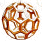xGraph theory
Confusing Concepts
Posts  1 - 1  of  1tarektarek
Hi all,
I want to make sure that I understood some concepts of graph theory.
I had a Theorem which generally states "The routing protocol guarantees packet delivery". See Theorem 1
in http://ieeexplore.ieee.org/stamp/stamp.jsptp=&arnumber=4654241&isnumber=4654185

Note: If you can not get it let me know.

So when I proved it, somebody told me that how did you prove it, so my answer was by contradiction, which gave me the impression that this is a bad answer.

After some readings, can I say that I proved it based on Geometric Graph Theory
where I am using for my Theorem "a Euclidean graph which is a graph in which the vertices represent points in the plane, and the edges are assigned lengths equal to the Euclidean distance between those points. " From http://en.wikipedia.org/wiki/Geometric_graph_theory

And then I will use the proof by contradiction to prove my protocol.
Is it what I should have said when I have been asked?